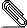[Wien] Spin-polarization VS no spin-polarization in GaN

Dear Sirs,

I did some calculations with GaN (struct file in attachment) to compare the
difference in energy and electric field gradient between the use of
spin-polarization or not.

The calculations were done using the commands

init_lapw -b -ecut -8 -numk 480 -rkmax 7 (-sp)
run(sp)_lapw -p -ec 0.00001 -cc 0.0001 -fc 0.5

and the results were the following

:ENE = -7995.60614872 (no spin-polarization)
:ENE = -7995.60614871 (spin-polarization)

:EFG001 = 0.57937 (no sp)
:EFG001 = 0.57931 (sp)

so they basically give the same.

Then, I relaxed the structure using

run(sp)_lapw -p -ec 0.00001 -cc 0.0001 -fc 0.5 -min

and the results were

:POS001 = 0.66667 0.33333 0.00002 (no sp)
:POS001 = 0.66667 0.33333 0.00000 (sp)

:POS002 = 0.66667 0.33333 0.37662 (no sp)
:POS002 = 0.66667 0.33333 0.37701 (sp)

:ENE = -7995.60615476 (no sp)
:ENE = -7995.60615035 (sp)

:EFG001 = 0.45038 (no sp)
:EFG001 = 0.58801 (sp)

so they gave different final positions and very different :EFG, as a
consequence.

However, if I use the final structure from the minimization without
spin-polarization and do a calculation with spin-polarization (both from the
beginning, using the previous commands), I get equal values

:ENE = -7995.60615467 (no sp)
:ENE = -7995.60615466 (sp)

:EFG001 = 0.45036 (no sp)
:EFG001 = 0.45034 (sp)

and if I use the final structure from the minimization with spin-polarization
and do a calculation without spin-polarization, I also get equal values

:ENE = -7995.60614842 (no sp)
:ENE = -7995.60614842 (sp)

:EFG001 = 0.58272 (no sp)
:EFG001 = 0.58271 (sp)

This means that the only thing that I am getting different between them is the
atomic positions during the minimization, since I always get the same :ENE and
:EFG if both calculations are done with the same struct file.

Is there any reason for this to happen?
Which values for :ENE, :EFG and :POS should I consider the best, the
spin-polarized ones or the ones without spin-polarization?

Best regards,
MarceloGaN.struct
Description: Binary data

_______________________________________________
Wien mailing list
Wien@zeus.theochem.tuwien.ac.at
http://zeus.theochem.tuwien.ac.at/mailman/listinfo/wien
SEARCH the MAILING-LIST at:
http://www.mail-archive.com/wien@zeus.theochem.tuwien.ac.at/index.html